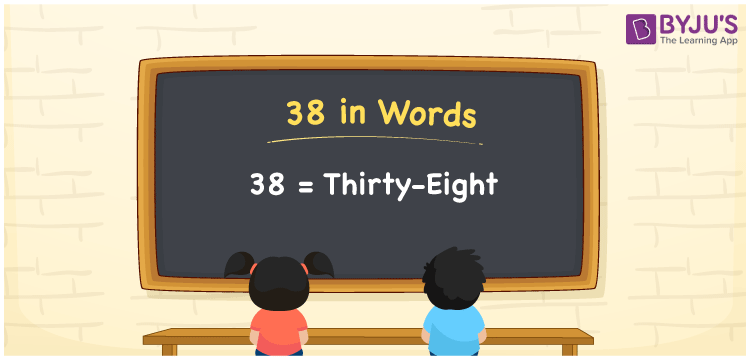# 38 in Words

38 in words is written as “Thirty-eight”. In Mathematics, 38 is a natural number that is used to represent a value or a quantity. For example, If Johnson has Thirty-eight marbles. Also, Rs.38 can be written as Rupees Thirty-eight. Hence, 38 in words is used in different ways in Maths and in real life. Learn more about Numbers In Words at BYJU’S.

 38 in Words Thirty-eight Thirty-eight in Numbers 38

## 38 in English Words

38 in Words using English alphabets is expressed as given below:## How to Write 38 in Words?

By knowing the place values of each digit in a number, we can name the number or write it in words. To write 38 in words, let us see the positions of each digit in the below table.

 Tens Ones 3 8

From the above table, we can see, 3 is at tens place (30) and 8 is at ones place (8). We need to read the number from right to left, in the table. Thus, together we can write the number, thirty and eight, as Thirty-eight.

### Expanded Form of 38

We can write the expanded form of 38 as:

3 at Tens + 8 at Ones

= 3 × 10 + 8 × 1

= 38

= Thirty-eight

38 is a whole number that is succeeded by 37 and preceded by 39. Find more about the number 38 below:

• 38 in Words – Thirty-eight
• Is 38 an odd number? – No
• Is 38 an even number? – Yes
• Is 38 a perfect square number? – No
• Is 38 a perfect cube number? – No
• Is 38 a prime number? – No
• Is 38 a composite number? – Yes

## Frequently Asked Questions on 38 in words

Q1

### What is 38 in words?

38 in words is given by Thirty-eight.
Q2

### What is the rule to write 38 in words?

38 in words is written based on the place values of each digit. In 38, 3 is at tens place and 8 is at units place. Thus, thirty plus eight is read as Thirty-eight.
Q3

### How to write 38 in English words?

38 in English is written as Thirty-eight